## research papersJOURNAL OFSYNCHROTRONRADIATION
ISSN: 1600-5775
Volume 24| Part 4| July 2017| Pages 796-801

## X-ray reflectivity measurement of interdiffusion in metallic multilayers during rapid heating

aDepartment of Materials Science and Engineering, Johns Hopkins University, Baltimore, MD 21218, USA, bSchool of Materials Science and Engineering, Beijing Institute of Technology, Beijing 100081, People's Republic of China, cCornell High Energy Synchrotron Source (CHESS), Cornell University, Ithaca, NY 14853, USA, dDepartment of Physics, Cornell University, Ithaca, NY 14853, USA, and eKavli Institute at Cornell for Nanoscale Science, Cornell University, Ithaca, NY 14853, USA
*Correspondence e-mail: hufnagel@jhu.edu

(Received 16 December 2016; accepted 30 May 2017; online 15 June 2017)

A technique for measuring interdiffusion in multilayer materials during rapid heating using X-ray reflectivity is described. In this technique the sample is bent to achieve a range of incident angles simultaneously, and the scattered intensity is recorded on a fast high-dynamic-range mixed-mode pixel array detector. Heating of the multilayer is achieved by electrical resistive heating of the silicon substrate, monitored by an infrared pyrometer. As an example, reflectivity data from Al/Ni heated at rates up to 200 K s−1 are presented. At short times the interdiffusion coefficient can be determined from the rate of decay of the reflectivity peaks, and it is shown that the activation energy for interdiffusion is consistent with a grain boundary diffusion mechanism. At longer times the simple analysis no longer applies because the evolution of the reflectivity pattern is complicated by other processes, such as nucleation and growth of intermetallic phases.

### 1. Introduction

Solid-state interdiffusion is of profound importance in nanostructured materials, where the diffusion distances are short and diffusion times can be small. In semiconductor electronics, for example, the possibility of device failure resulting from interdiffusion has spurred extensive research into materials that can act as diffusion barriers between device components (Nicolet, 1997). Because common analysis techniques either require destructive depth profiling or are rather slow, most studies of interdiffusion are performed ex situ, often after isothermal annealing. Such studies usually assume that the transients associated with heating and cooling the specimen can be neglected.

In some situations, however, the transients themselves are of interest, for example in rapid thermal annealing. In other situations, interfacial reactions alter the structure of the material in ways that make the interpretation of interdiffusion measurements after the fact difficult or impossible. It is therefore desirable to develop experimental techniques that allow measurements in situ, while interdiffusion is occurring.

One such technique is X-ray reflectivity (low-angle diffraction) performed on multilayer materials. In the low-angle region the intensities of X-ray scattering peaks are related to the composition modulations through the thickness of the multilayer. By monitoring the change in the intensities of these peaks we can measure interdiffusion. In fact, X-ray reflectivity is among the most sensitive probes of interdiffusion, capable of measuring interdiffusion coefficients as low as 10−27 m2 s−1 (Greer, 1997).

In a conventional X-ray reflectivity measurement the scattered intensity is recorded by step-scanning through a range of angles. This makes in situ observations impractical except for slow processes. To overcome this limitation, several techniques have been developed for recording complete reflectivity patterns simultaneously. For example, using a curved specimen one can record the scattering over a range of angles simultaneously using a position-sensitive X-ray detector (Naudon et al., 1989; Niggemeier et al., 1997; Stoev & Sakurai, 2013). Another approach is to use an X-ray beam with a range of wavelengths, either by dispersing the X-ray beam into a range of angles (Matsushita et al., 2008) (again using a position-sensitive detector) or, at a single angle, recording scattering with an energy-sensitive detector (Neissendorfer et al., 1999; Raghavendra Reddy et al., 2009).

In this paper we show how to use the curved-sample approach to perform time-resolved in situ X-ray reflectivity characterization of the initial stages of interdiffusion during continuous heating of metallic multilayers. We have tested our technique at heating rates up to 200 K s−1, but in principle it can be applied at much higher rates, limited by the intensity of the X-ray source and the capabilities of the X-ray detector. During the initial stages of heating we can determine the interdiffusion coefficient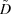by a simple analysis of the rate of decay of the peaks in the reflectivity pattern, which are related to the composition modulation of the multilayer. At longer times this simple analysis becomes unreliable because the reflectivity pattern is affected by other processes, such as nucleation and growth of intermetallic phases.

### 2. Experimental

The samples for this study were multilayer foils produced by DC magnetron sputtering alternating layers of alluminum alloy 1100 with layers of nickel–7 wt% vanadium. The ratio of the Al layer thickness to the Ni–V layer thickness was 3:2, which yields an atomic ratio of Al:Ni–V of 1:1. The bilayer period of the layers (i.e. the sum of one Al and one Ni–V layer thickness) was 20–30 nm. Because X-ray reflectivity is strongly sensitive to the roughness of the layers and the roughness increases with the number of layers deposited, we restricted the thickness of our samples to three to six bilayer periods (Al/Ni layer pairs). The multilayers were deposited onto 500 µm-thick polished Si wafers, onto which we also deposited 300 nm-thick gold pads by thermal evaporation (Fig. 1a). (A 20 nm-thick layer of tungsten was deposited first to promote adhesion of the gold to the silicon substrate.) The gold pads provided electrical contacts to permit resistive heating of the doped Si substrate, which in turn heated the multilayer. The power source for these experiments was a series array of 9 V or 12 V batteries, switched with a solid-state relay to allow control of the duration of the current pulse. We monitored the temperature of the multilayer during heating with a single-wavelength infrared pyrometer (Kleiber KGA 740-LO), sampling at 50 Hz. The lowest temperature that can be measured with this pyrometer is 475 K, so we were unable to use it to track the very earliest stages of heating.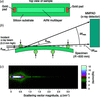Figure 1 (a) The samples had lithographically patterned regions of Al/Ni multilayer (4 cm long by 1 cm wide) along with gold pads (0.5 cm by 1 cm) to act as electrical contacts. (b) The samples were dynamically bent in a four-point loading apparatus, with the curvature of the specimen measured by a laser wafer-curvature technique and temperature monitored by an optical pyrometer. (c) Example raw data from a 3 × 1 section of chips on the MMPAD array.

Angle-dispersive X-ray reflectivity uses curved specimens so that the angle of incidence of X-rays on the surface varies with position, as shown in Fig. 1(b). Although multilayers can be deposited on curved substrates, we elected instead to use flat substrates which we then bent in a specially designed loading fixture. Pragmatically, flat substrates are cheaper than precisely polished curved substrates, and it is easier to deposit uniform multilayers on them. This approach also allows flexibility in choosing the radius of curvature (and thus the range of angles over which the reflectivity is measured). Our four-point bending apparatus is illustrated in Fig. 1(b). The two lower loading rods are fixed in position, and bending is achieved by using stepper motors to displace the two upper rods. Gaps in the upper rods (not shown) provide a clear path for the X-rays, and the rods (which are made of steel) are electrically isolated from the substrate by kapton tape. Prior to each reflectivity measurement we measured the curvature of the specimen by means of a parallel-beam curvature setup similar to that described by Floro et al. (1996).

The experiments described here were performed at station A2 of the Cornell High Energy Synchrotron Source (CHESS) using a Si(111) double-crystal monochromator to select 12.0 keV X-rays, with a flux of approximately 5 × 1010 photons s−1 mm−2. The beam height (0.3 mm) yielded a range of incident angles from zero to approximately 1.3°, depending on the radius of curvature of the specimen (typically about 500 mm). The X-ray detector was a mixed-mode pixel array detector (MMPAD), which is capable of framing continuously at high rates (up to 1 kHz) and has a large dynamic range (>4 × 107 photons pixel−1) (Tate et al., 2013). The dynamic range is useful because it allows both the low- and high-order scattering peaks, which can differ in intensity by many orders of magnitude, to be measured simultaneously. The detector used here employed a 3 × 2 array of MMPAD chips (with each chip having a square 128 × 128 array of 150 µm pixels) although only a single row of three chips was used for these measurements (Fig. 1c). With this detector placed 1013 mm from the sample we recorded scattering over a range of scattering vectors (q =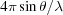, where θ is one-half of the scattering angle and λ is the X-ray wavelength) of about 3 nm−1. The width of the X-ray beam was 1 mm, and the one-dimensional reflectivity patterns shown below were obtained by simply summing the output from the detector across the width of the beam at each row of pixels corresponding to a given value of q.

Fig. 2shows X-ray reflectivity data from an Al/Ni multilayer sample with a nominal bilayer period of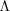= 20 nm, recorded two ways: using the apparatus described above, and on a conventional parallel-beam diffractometer (Philips MRD) using Cu Kα radiation with the sample nominally flat. Although the basic features of the laboratory reflectivity data are reproduced in the in situ synchrotron experiment, the agreement is not perfect. There are several reasons for this. First, the in situ technique records some non-specular scattering (θincidentθexit) in addition to the desired specular scattering (θincident = θexit). Second, there is variation in the incident intensity in the in situ case due to the intensity profile along the height of the synchrotron beam. Third, no attempt has been made to correct for geometrical aberrations such as anticlastic bending of the substrate. Finally, the energy bandpass and the angular divergence of the X-ray beam are different between the two cases.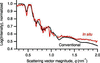Figure 2 Comparison of reflectivity profiles measured with the in situ apparatus and a conventional laboratory diffractometer. The intensities have been normalized to match in the total external reflection region at small q. Here, and in the other figures, log refers to base-10 logarithm while ln refers to natural (base e) logarithm

Despite these differences the two reflectivity profiles are in reasonably good agreement. Both profiles show an intensity plateau below about q = 0.4 nm−1 which is due to total external reflection of X-rays from the multilayer. Above this, both profiles show a series of low-angle scattering peaks from the multilayer structure.

### 3. Results and discusson

Fig. 3(a)shows reflectivity data from an Al/Ni multilayer recorded in situ during heating at 40 K s−1. The intensity of the first peak (shown in the inset) decreases with increasing temperature. The amplitudes of the peaks in the low-angle region are proportional to the square of the amplitude of the composition modulation (Cook, 1969; Paulson & Hilliard, 1977). If no phase transformation occurs (a point to which we return below) as interdiffusion proceeds, the composition of the multilayer becomes more uniform and the intensity of the low-angle peaks decreases.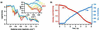Figure 3 (a) Evolution of the X-ray reflectivity of an Al/Ni multilayer with nominal bilayer period= 25 nm during heating at 40 K s−1. Each exposure was 100 ms. The inset shows the first low-angle scattering peak in more detail. (b) Evolution of peak intensity, plotted as ln[I(T)/I(473 K)], with time for determination of.

For the more common case of interdiffusion studied at constant temperature, the intensity of a low-angle scattering peak I(t) at time t is related to that at initial time t0 by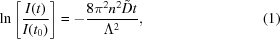where n is the order of the reflection andis the interdiffusion coefficient (Wang et al., 1999). The bulk interdiffusion coefficientcan therefore be determined from the slope of a plot of ln[I(t)/I(t0)] against t.

There are several issues with applying equation (1)to the present case. First, our experiments were conducted at constant heating rate. We make the assumption that because our heating rates are high andincreases exponentially with temperature, the amount of interdiffusion that occurs at temperature T over a given interval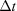is large compared with the amount of interdiffusion that occurred in heating up to that temperature. This assumption is easily checked by comparing the integral of the diffusion equation for constant heating rate with that for isothermal interdiffusion (given the activation energy for interdiffusion) (Khawam & Flanagan, 2006). This assumption allows us to recast equation (1)in terms of temperature and write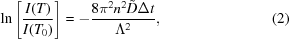whereis the interval between measurements (100 ms for the experiments described here). Fig. 3(b)shows a plot of ln[I(T)/I(T0)] against t, for T0 = 473 K.

Another potential complication in the application of equation (1)for measuring interdiffusion in multilayers is that it does not apply to situations in which the concentration gradients are very steep. As discussed by Greer & Spaepen (1985), however, this effect is small when the bilayer period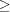6d, where d is the atomic spacing parallel to the diffusion direction. The interplanar spacings of Al and Ni are around 2.0–2.3 Å [for the Ni(111) and Al(111) planes, respectively], much smaller than the bilayer periods used here (20–30 nm). Furthermore, the substantial intermixing that occurs during sputter deposition of Al/Ni multilayers (Gavens et al., 2000) acts to reduce the concentration gradient. Therefore, equation (1)can be applied without an explicit correction for the effect of the concentration gradient.

Fig. 4(a)shows the interdiffusion coefficientas a function of temperature, determined from the decay of the low-angle scattering peaks using equation (2), for several combinations of bilayer period and heating rates. We begin by focusing our attention on the low-temperature end of Fig. 4(a). If the evolution of the composition profile is dominated by a single thermally activated diffusion mechanism, thenshould increase exponentially with temperature. To check this, Fig. 4(b)shows an Arrhenius plot of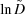versus 1/T for the= 25 nm multilayer heated at 40 K s−1. We see that at the lowest temperatures the behavior is indeed linear, with an apparent activation energy for interdiffusion of Ea = 92 ± 7 kJ mol−1. Data for the= 30 nm multilayer heated at 200 K s−1 yield a similar value, Ea = 80 ± 19 kJ mol−1. We were not able to extract a reliable activation energy for the smallest bilayer period (= 20 nm) due to substantial interdiffusion that occurred during heating up to the lowest temperature at which we could make reliable pyrometer measurements (475 K).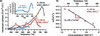Figure 4 (a) Interdiffusion coefficientfor several combinations of bilayer period and heating rate. Labels A, B and C identify the peaks infor the multilayer with= 25 nm discussed in the main text and referenced in Fig. 5. The inset shows the data for= 20 nm in more detail. (b) Arrhenius plot for determination of activation energy Ea for the= 25 nm sample from part (a). The fit is to the five data points from the lowest temperature range (485 K to 530 K).

Du and co-workers (Du et al., 2003) performed a critical assessment of bulk interdiffusion coefficients in a variety of systems and reported an activation energy of Ea = 144.6 kJ mol−1 for diffusion of Ni in face-centered-cubic Al, based on indirect observations by Erdélyi and co-workers (Erdélyi et al., 1978). If we assume that interdiffusion is dominated by diffusion of nickel [because nickel is known to be a fast diffuser in aluminium (Edelstein et al., 1994)], as a rough approximation we may also take this value of activation energy as representative of interdiffusion. For Ni-rich alloys, Watanabe and co-workers reported higher activation energies for interdiffusion, Ea = 214–277 kJ mol−1 (Watanabe et al., 1994). Taken together, this prior work, though limited, suggests an activation energy for interdiffusion of roughly Ea = 200 ± 50 kJ mol−1.

However, those earlier measurements were from higher temperatures [742–924 K (Erdélyi et al., 1978) and 1050–1400 K (Watanabe et al., 1994)] than those we used for our determination of Ea (Fig. 4b). It is reasonable to suspect that grain boundary diffusion may dominate at lower temperatures, particularly for our multilayers where the grain size is of the order of a few nanometers. If we assume that this is the case, and that the activation energy for grain boundary diffusion is about half that for lattice diffusion [based on an average value for face-centered-cubic metals (Brown (1980)], we arrive at a value of around 100 ± 25 kJ mol−1. This is consistent with recent measurements (at temperatures similar to ours) by Grieseler and co-workers who reported Ea = 120 kJ mol−1 and also assumed a grain boundary diffusion mechanism (Grieseler et al., 2014). Another point of comparison is an activation energy for solid-state interdiffusion of 77 ± 1 kJ mol−1 calculated by Fritz and co-workers based on the ignition threshold for self-propagating reactions in Al/Ni–V multilayers very similar to those considered here (Fritz et al., 2013). All of these numbers are reasonably consistent with our measured values of ∼90 kJ mol−1.

After this initial stage of interdiffusion during whichincreases exponentially with temperature, more complex behavior is observed at higher temperatures (Fig. 4a). This complexity presumably results from other processes that also affect the composition profile of the multilayer. Al/Ni multilayers undergo a series of intermetallic formation reactions with increasing temperature, the details of which depend on the overall composition, bilayer period and heating rate (Knepper et al., 2009; Grapes et al., 2014). Activation energies for phase transformations occurring at constant heating rate are commonly determined using the Kissinger equation,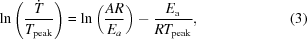where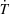is the heating rate, Tpeak is the temperature at the peak maximum in a DSC scan, A is a pre-exponential constant, R is the gas constant and Ea is the activation energy. Data drawn from Grapes (2016) for the formation of Al3Ni, Al3Ni2 and AlNi at various heating rates are shown in Fig. 5. Also indicated on this plot are the temperatures of the three peaks inlabeled A, B and C in Fig. 4(a)for the= 25 nm sample heated at 40 K s−1. Peaks B and C appear at temperatures close to those expected for the formation of Al3Ni and Al3Ni2, respectively. The decrease in apparentabove these temperatures may or may not be real. The intermetallic phases act as diffusion barriers and reduce, although we note that these phases also have grain boundaries which would reduce their effectiveness as diffusion barriers. Alternatively, the formation of the intermetallic phases may affect the reflectivity pattern in ways that only make it appear thatis decreasing.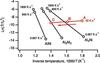Figure 5 Kissinger plots for the formation of the intermetallic phases Al3Ni, Al3Ni2 and AlNi, based on DSC data from Grapes (2016). Points A, B and C refer to the temperatures at which peaks inoccur for the= 25 nm sample (Fig. 4a). Joining them is a line indicating a heating rate of 40 K s−1; the arrow indicates the direction of increasing temperature along this line. At this heating rate, peak B occurs at a temperature close to that which would be expected for formation of Al3Ni and peak C near the temperature expected for Al3Ni2.

Peak A, on the other hand, occurs at a lower temperature than would be expected for formation of any intermetallic phase at this heating rate. In our view, this behavior most likely results from the composition dependence of. In particular, if grain boundary diffusion dominates at low temperatures then it may be that the Al grain boundaries quickly become saturated with Ni, which would slow down subsequent diffusion.

### 4. Conclusions

We have demonstrated an X-ray reflectivity technique for measuring solid-state interdiffusion in multilayer materials during rapid heating. Here we have demonstrated the technique at rates of up to 200 K s−1 but it could readily be extended to higher rates. We have, for example, collected reflectivity patterns with reasonably good signal-to-noise ratios in as little as 2 ms, implying the ability to collect data at heating rates as fast as 104 K s−1. More detailed studies, for example of interlayer roughness, may also be possible from a more complete consideration of the two-dimensional reflectivity profiles (Fig. 1). This would require careful corrections for the intensity profile of the incident beam and geometrical aberrations due to the curved sample (Stoev & Sakurai, 2013), along with modeling of the specular and diffusion scattering from the specimens.

### Footnotes

Present address: Joint Center for Artificial Photosynthesis, California Institute of Technology, Pasadena, CA 91125, USA.

### Acknowledgements

JK, MZ, LZ, TPW and TCH gratefully acknowledge support for this work from the US Department of Energy under grant No. DE-SC002509. JPL gratefully acknowledges financial support from the Chinese Scholarship Council (CSC). This work is based upon research conducted at the Cornell High Energy Synchrotron Source (CHESS) which is supported by the National Science Foundation and the National Institutes of Health/National Institute of General Medical Sciences under NSF award DMR-1332208. Detector development at Cornell is supported by the DOE Grant No. DE-SC0016035 and CHESS.

### References

This is an open-access article distributed under the terms of the Creative Commons Attribution (CC-BY) Licence, which permits unrestricted use, distribution, and reproduction in any medium, provided the original authors and source are cited.JOURNAL OFSYNCHROTRONRADIATION
ISSN: 1600-5775
Volume 24| Part 4| July 2017| Pages 796-801